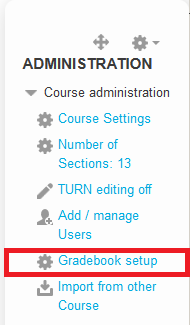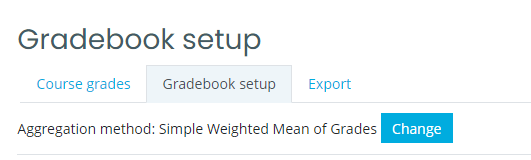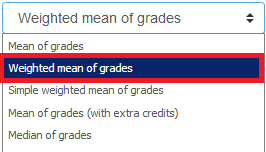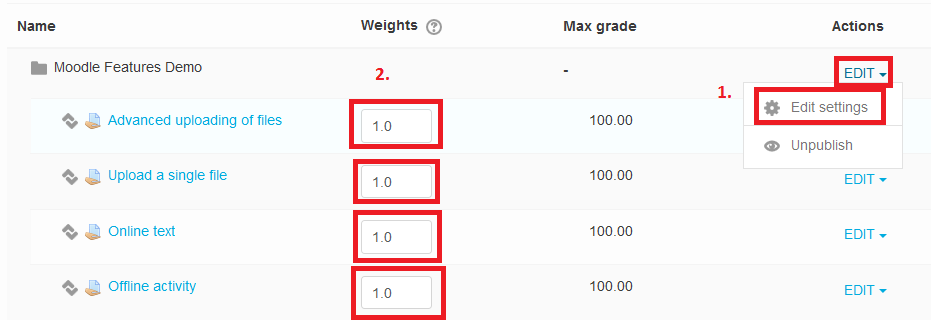### Create Activities (give tasks to students)

#### How to use and modify weights in the gradebook?The Gradebook in Moodle can help you organize and manage grades in your course.

• Students can view their own grades at one place.
• When grades are awarded from within a Moodle activity (assignment), scores are automatically added to the gradebook.
• Scores can also be manually added or edited (Overridden) in the gradebook.

In order for Moodle to easily aggregate scores automatically, entries in the gradebook are numeric by default. The default score for each activity is 100. You can then control the weights of individual activities to aggregate a final score for the class. If you prefer to grade only with letters no numeric scores are involved and Moodle cannot aggregate a final grade for the course.

Default calculation of the final grade in Moodle - Simple weighted mean or weighted mean.
If you would like to give extra credits
(see below), use Natural.

For simple weighted main, the weight of each item is simply the difference between its Maximum and Minimum grade.

Example:

A1 70/100, A2 20/80, A3 10/10, category max 100:
(0.7*100 + 0.25*80 + 1.0*10)/(100 + 80 + 10) = 0.526 --> 52.6/100
If you would like to adjust the weights of individual assignments, you need to change gradebook calculation method to "weighted mean of grades".

1.
Go to the Gradebook, and select the "Gradebook setup" menu.2.
Click on the "change" link next to the aggregation method, and change the "simple weighted mean" to "weighted mean of grades" and save.3. For "weighted mean of grades" adjust the weights next to individual assignments.So it will work like this:

Each grade item can be given a weight to influence its importance in the overall mean. In simple terms, the "total" will be equal to the sum of the scores in each grade item, these scores being multiplied by the grade items' weights, and that sum being finally divided by the sum of the weights, as shown in this example.

Example:A1 70/100 weight 10, A2 20/80 weight 5, A3 10/10 weight 3, category max 100:
(0.7*10 + 0.25*5 + 1.0*3)/(10 + 5 + 3) = 0.625 --> 62.5/100

4. How to give extra credits for any assignment or task

How to set extra credits?
You need to use the Natural aggregation method.
To set an item to add extra credits instead of normal grading click on EDIT > edit settings next to the item.
Tick the "extra credit" box and save.